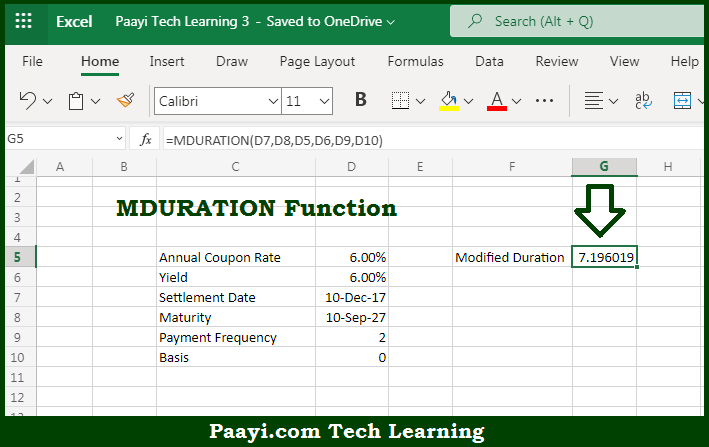# Learn How to Use Microsoft Excel MDURATION Function

Written by | 0 Comments | 483 Views

In this article, you will learn how to use the Microsoft Excel MDURATION function and its prime function in Microsoft Excel. You will also get to know the Microsoft Excel MDURATION function return value and syntax with the help of some examples.

Microsoft Excel MDURATION Function

The main purpose of the Microsoft Excel MDURATION function is to return the Macauley modified duration for a security with an assumed par value of \$100. That implies, with the help of MDURATION function you can able to get the Macauley modified duration for a given security which is having a par value of \$100 as an assumption. So, with the help of MDURATION function, you can able to get the Macauley modified duration for a security with an assumed par value of \$100.

Return Value of MDURATION Function

The return value will be the modified duration in years.

Syntax of MDURATION Function

=MDURATION(settlement, maturity, coupon, yld, freq, [basis])

Where the arguments:

• settlement: This is the settlement date of the security.
• maturity: This is the maturity date of the security.
• coupon: This is the annual coupon rate of the security.
• yld: This annual yield of the security.
• freq: This is the number of coupon payments per year, where annual = 1, semi-annual = 2, quarterly = 4.
• basis: This is the day count basis, defaults to 0 (optional).

How to Use Microsoft Excel MDURATION Function?So we know that Microsoft Excel MDURATION function you can able to return the Macauley modified duration for a security with an assumed par value of \$100. That implies, with the help of MDURATION function you can able to get the Macauley modified duration for given security which is having a par value of \$100 as an assumption. So, with the help of MDURATION function, you can able to get the Macauley modified duration for a security with an assumed par value of \$100.